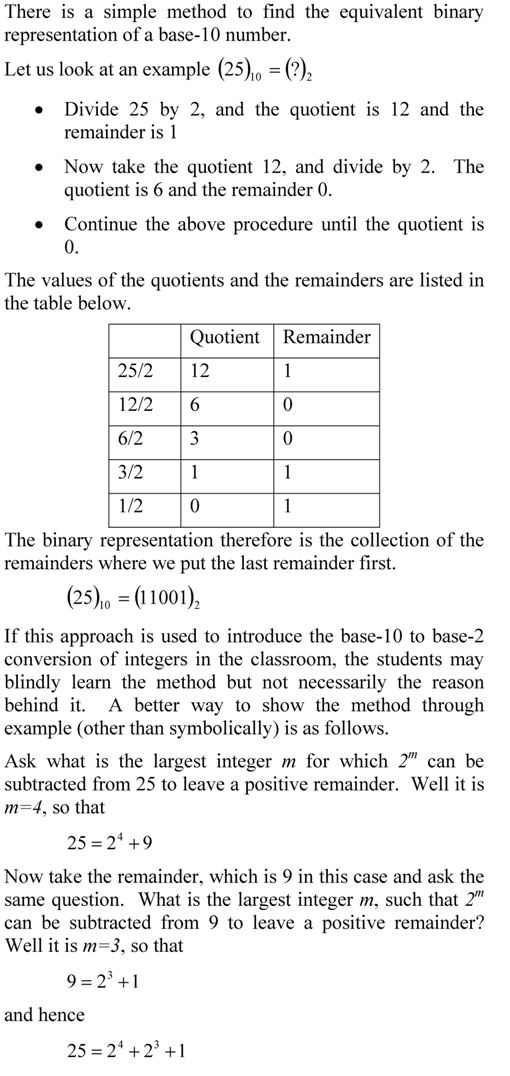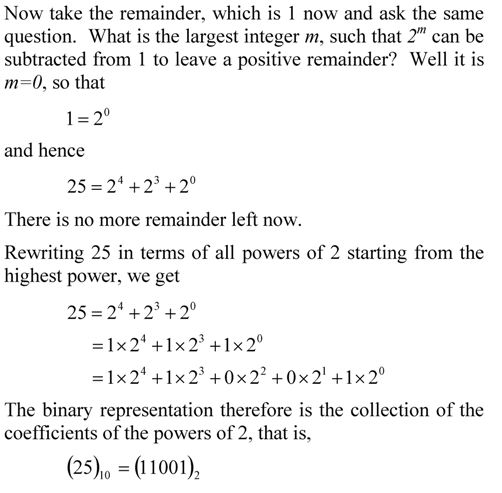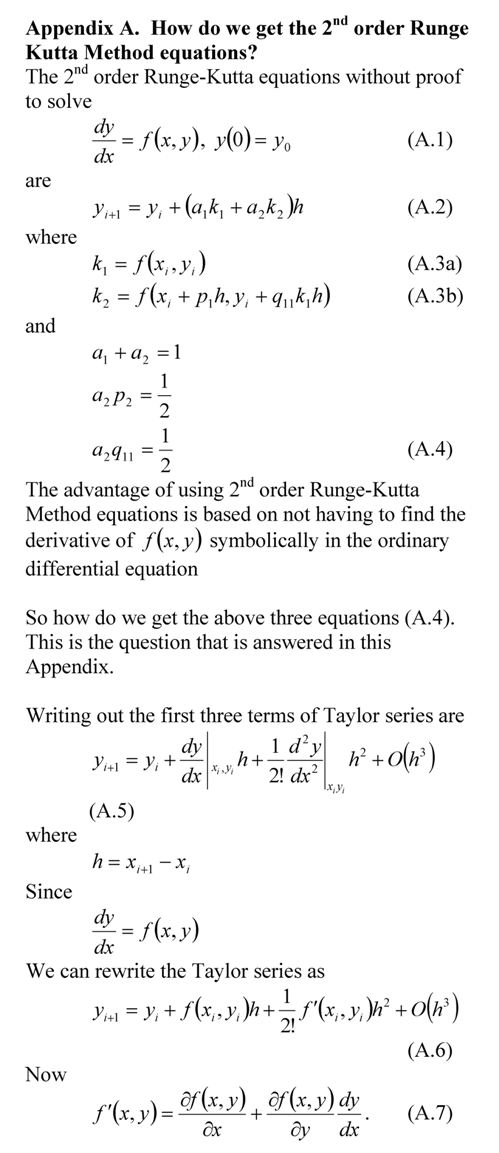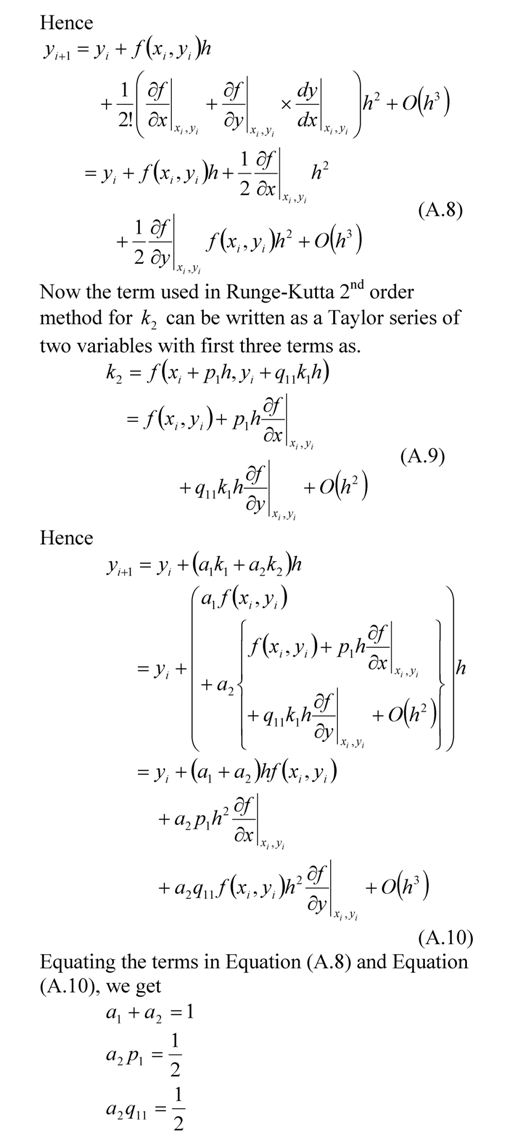## A better way to show decimal to binary conversionThis post is brought to you by Holistic Numerical Methods: Numerical Methods for the STEM undergraduate at http://numericalmethods.eng.usf.edu.

An abridged (for low cost) book on Numerical Methods with Applications will be in print (includes problem sets, TOC, index) on December 10, 2008 and available at lulu storefront.

## Accuracy of Taylor series

So how many terms should I use in getting a certain pre-determined accuracy in a Taylor series. One way is to use the formula for the Taylor’s theorem remainder and its bounds to calculate the number of terms. This is shown in the example below.This post is brought to you by Holistic Numerical Methods: Numerical Methods for the STEM undergraduate at http://numericalmethods.eng.usf.edu.

An abridged (for low cost) book on Numerical Methods with Applications will be in print (includes problem sets, TOC, index) on December 10, 2008 and available at lulu storefront.

## Taylor series example

If Archimedes were to quote Taylor’s theorem, he would have said, “Give me the value of the function  and the value of all (first, second, and so on) its derivatives at a single point, and I can give you the value of the function at any other point”.

It is very important to note that the Taylor’s theorem is not asking for the expression of the function and its derivatives, just the value of the function and its derivatives at a single point.

Now the fine print: Yes, all the derivatives have to exist and be continuous between x and x+h, the point where you are wanting to calculate the function at. However, if you want to calculate the function approximately by using the nth order Taylor polynomial, then 1st, 2nd,…., nth derivatives need to exist and be continuous in the closed interval [x,x+h], while the (n+1)th derivative needs to exist and be continuous in the open interval (x,x+h).## Taylor Series Revisited

Taylor series is a very important concept that is used in numerical methods. From the concept of truncation error to finding the true error in Trapezoidal rule, having a clear understanding of Taylor series is extremely important. Other places in numerical methods where Taylor series concept is used include: the derivation of finite difference formulas for derivatives, finite difference method of solving differential equations, error in Newton Raphson method of solving nonlinear equations, Newton divided difference polynomial for interpolation, etc.

I have written a short chapter on Taylor series. After reading the chapter, you should be able to:

1. understand the basics of Taylor’s theorem,

2. see how transcendental and trigonometric functions can be written as Taylor’s polynomial,

3. use Taylor’s theorem to find the values of a function at any point, given the values of the function and all its derivatives at a particular point,

4. errors and error bounds of approximating a function by Taylor series,

5. revisit the chapter whenever Taylor’s theorem is used to derive or explain numerical methods for various mathematical procedures.

This post is brought to you by Holistic Numerical Methods: Numerical Methods for the STEM undergraduate at http://numericalmethods.eng.usf.edu.

An abridged (for low cost) book on Numerical Methods with Applications will be in print (includes problem sets, TOC, index) on December 10, 2008 and available at lulu storefront.

## Runge-Kutta 2nd order equations derived

In my class, I present the 2nd order Runge-Kutta method equations without proof. Although I do discuss where the equations come from, there are still students who want to see the proof. So here it is._____________________________________________________

This post is brought to you by Holistic Numerical Methods: Numerical Methods for the STEM undergraduate at http://numericalmethods.eng.usf.edu

## A Matlab program for comparing Runge-Kutta methods

In a previous post, we compared the results from various 2nd order Runge-Kutta methods to solve a first order ordinary differential equation. In this post, I am posting the matlab program. It is better to download the program as single quotes in the pasted version do not translate properly when pasted into a mfile editor of MATLAB or see the html version for clarity and sample output .

Do your own testing on a different ODE, a different value of step size, a different initial condition, etc. See the inputs section below that is colored in bold brick.

% Simulation : Comparing the Runge Kutta 2nd order method of
% solving ODEs
% Language : Matlab 2007a
% Authors : Autar Kaw
% Last Revised : July 12, 2008
% Abstract: This program compares results from the
% exact solution to 2nd order Runge-Kutta methods
% of Heun’s method, Ralston’s method, Improved Polygon
% method, and directly using the three terms of Taylor series
clc
clear all
clc
clf
disp(‘This program compares results from the’)
disp(‘exact solution to 2nd order Runge-Kutta methods’)
disp(‘of Heuns method, Ralstons method, Improved Polygon’)
disp(‘ method, and directly using the three terms of Taylor series’)

%INPUTS. If you want to experiment these are only things
% you should and can change. Be sure that the ode has an exact
% solution
% Enter the rhs of the ode of form dy/dx=f(x,y)
fcnstr=’sin(5*x)-0.4*y’ ;
% Initial value of x
x0=0;
% Initial value of y
y0=5;
% Final value of y
xf=5.5;
% number of steps to go from x0 to xf.
% This determines step size h=(xf-x0)/n
n=10;

%REST OF PROGRAM
%Converting the input function to that can be used
f=inline(fcnstr) ;

% EXACT SOLUTION
syms x
eqn=[‘Dy=’ fcnstr]
% exact solution of the ode
exact_solution=dsolve(eqn,’y(0)=5′,’x’)
% geting points for plotting the exact solution
xx=x0:(xf-x0)/100:xf;
yy=subs(exact_solution,x,xx);
yexact=subs(exact_solution,x,xf);
plot(xx,yy,’.’)
hold on

% RUNGE-KUTTA METHODS
h=(xf-x0)/n;
% Heun’s method
a1=0.5;
a2=0.5;
p1=1;
q11=1;
xr=zeros(1,n+1);
yr=zeros(1,n+1);
%Initial values of x and y
xr(1)=x0;
yr(1)=y0;
for i=1:1:n
k1=f(xr(i),yr(i));
k2=f(xr(i)+p1*h,yr(i)+q11*k1*h);
yr(i+1)=yr(i)+(a1*k1+a2*k2)*h;
xr(i+1)=xr(i)+h;
end
%Value of y at x=xf
y_heun=yr(n+1);
% Absolute relative true error for value using Heun’s Method
et_heun=abs((y_heun-yexact)/yexact)*100;
hold on
xlabel(‘x’)
ylabel(‘y’)
title_name=[‘Comparing exact and Runge-Kutta methods with h=’ num2str(h)] ;
title(title_name)
plot(xr,yr, ‘color’,’magenta’,’LineWidth’,2)
% Midpoint Method (also called Improved Polygon Method)
a1=0;
a2=1;
p1=1/2;
q11=1/2;
%Initial values of x and y
xr(1)=x0;
yr(1)=y0;
for i=1:1:n
k1=f(xr(i),yr(i));
k2=f(xr(i)+p1*h,yr(i)+q11*k1*h);
yr(i+1)=yr(i)+(a1*k1+a2*k2)*h;
xr(i+1)=xr(i)+h;
end
%Value of y at x=xf
y_improved=yr(n+1);
% Absolute relative true error for value using Improved Polygon Method
et_improved=abs((y_improved-yexact)/yexact)*100;
hold on
plot(xr,yr,’color’,’red’,’LineWidth’,2)

% Ralston’s method
a1=1/3;
a2=2/3;
p1=3/4;
q11=3/4;
xr(1)=x0;
yr(1)=y0;
for i=1:1:n
k1=f(xr(i),yr(i));
k2=f(xr(i)+p1*h,yr(i)+q11*k1*h);
yr(i+1)=yr(i)+(a1*k1+a2*k2)*h;
xr(i+1)=xr(i)+h;
end
%Value of y at x=xf
y_ralston=yr(n+1);
% Absolute relative true error for value using Ralston’s Method
et_ralston=abs((y_ralston-yexact)/yexact)*100;
hold on
plot(xr,yr,’color’,’green’,’LineWidth’,2)

% Using first three terms of the Taylor series
syms x y;
fs=char(fcnstr);
% fsp=calculating f'(x,y) using chain rule
fsp=diff(fs,x)+diff(fs,y)*fs;
%Initial values of x and y
xr(1)=x0;
yr(1)=y0;
for i=1:1:n
k1=subs(fs,{x,y},{xr(i),yr(i)});
kk1=subs(fsp,{x,y},{xr(i),yr(i)});
yr(i+1)=yr(i)+k1*h+1/2*kk1*h^2;
xr(i+1)=xr(i)+h;
end
%Value of y at x=xf
y_taylor=yr(n+1);
% Absolute relative true error for value using Taylor series
et_taylor=abs((y_taylor-yexact)/yexact)*100;
hold on
plot(xr,yr,’color’,’black’,’LineWidth’,2)
hold off
legend(‘exact’,’heun’,’midpoint’,’ralston’,’taylor’,1)

% THE OUTPUT
fprintf(‘\nAt x = %g ‘,xf)
disp(‘ ‘)
disp(‘_________________________________________________________________’)
disp(‘Method Value Absolute Relative True Error’)
disp(‘_________________________________________________________________’)
fprintf(‘\nExact Solution %g’,yexact)
fprintf(‘\nHeuns Method %g %g ‘,y_heun,et_heun)
fprintf(‘\nImproved method %g %g ‘,y_improved,et_improved)
fprintf(‘\nRalston method %g %g ‘,y_ralston,et_ralston)
fprintf(‘\nTaylor method %g %g ‘,y_taylor,et_taylor)
disp( ‘ ‘)

________________________________________________________________________________________________

This post is brought to you by Holistic Numerical Methods: Numerical Methods for the STEM undergraduate at http://numericalmethods.eng.usf.edu

## Example to show how numerical ODE solutions can be used to find integrals

In a previous post, I enumerated how we can use numerical ODE techniques like Euler and Runge-Kutta methods to find approximate value of definite integrals. Here is an example. Be sure to do the exercises at the end of the post to appreciate the procedure._____________________________________________________

This post is brought to you by Holistic Numerical Methods: Numerical Methods for the STEM undergraduate at http://numericalmethods.eng.usf.edu